# Contents

This vignette shows how MOFA can be used to disentangle the heterogeneity in single-cell DNA methylation and RNA expression (scMT) data.

Briefly, the data set consists of 87 mouse embryonic stem cells (mESCs), comprising of 16 cells cultured in ‘2i’ media, which induces a naive pluripotency state, and 71 serum-grown cells, which commits cells to a primed pluripotency state poised for cellular differentiation (Angermueller, 2016).

# 1 Load data and create MOFA object

The data is stored as a `MultiAssayExperiment` object. Notice that there are 4 views, one with normalized RNA expression assay and 3 views with DNA methylation information. Each DNA methylation view is a different genomic context (i.e. Enhancers, Promoters and CpG Islands) and each feature is an individual CpG site.

``````library(MultiAssayExperiment)
library(MOFA)
library(ggplot2)``````
``````data("scMT_data")
scMT_data``````
``````## A MultiAssayExperiment object of 4 listed
##  experiments with user-defined names and respective classes.
##  Containing an ExperimentList class object of length 4:
##   RNA expression: ExpressionSet with 5000 rows and 81 columns
##   Met Enhancers: ExpressionSet with 5000 rows and 83 columns
##   Met CpG Islands: ExpressionSet with 5000 rows and 83 columns
##   Met Promoters: ExpressionSet with 5000 rows and 83 columns
## Features:
##  experiments() - obtain the ExperimentList instance
##  colData() - the primary/phenotype DataFrame
##  sampleMap() - the sample availability DataFrame
##  `\$`, `[`, `[[` - extract colData columns, subset, or experiment
##  *Format() - convert into a long or wide DataFrame
##  assays() - convert ExperimentList to a SimpleList of matrices``````

First, we create the MOFA object:

``MOFAobject <- createMOFAobject(scMT_data)``
``## Creating MOFA object from a MultiAssayExperiment object...``
``MOFAobject``
``````## Untrained MOFA model with the following characteristics:
##   Number of views: 4
##   View names: RNA expression Met Enhancers Met CpG Islands Met Promoters
##   Number of features per view: 5000 5000 5000 5000
##   Number of samples: 87``````

The function `plotDataOverview` can be used to obtain an overview of the structure of the data:

``plotDataOverview(MOFAobject, colors=c("#31A354","#377EB8","#377EB8","#377EB8"))``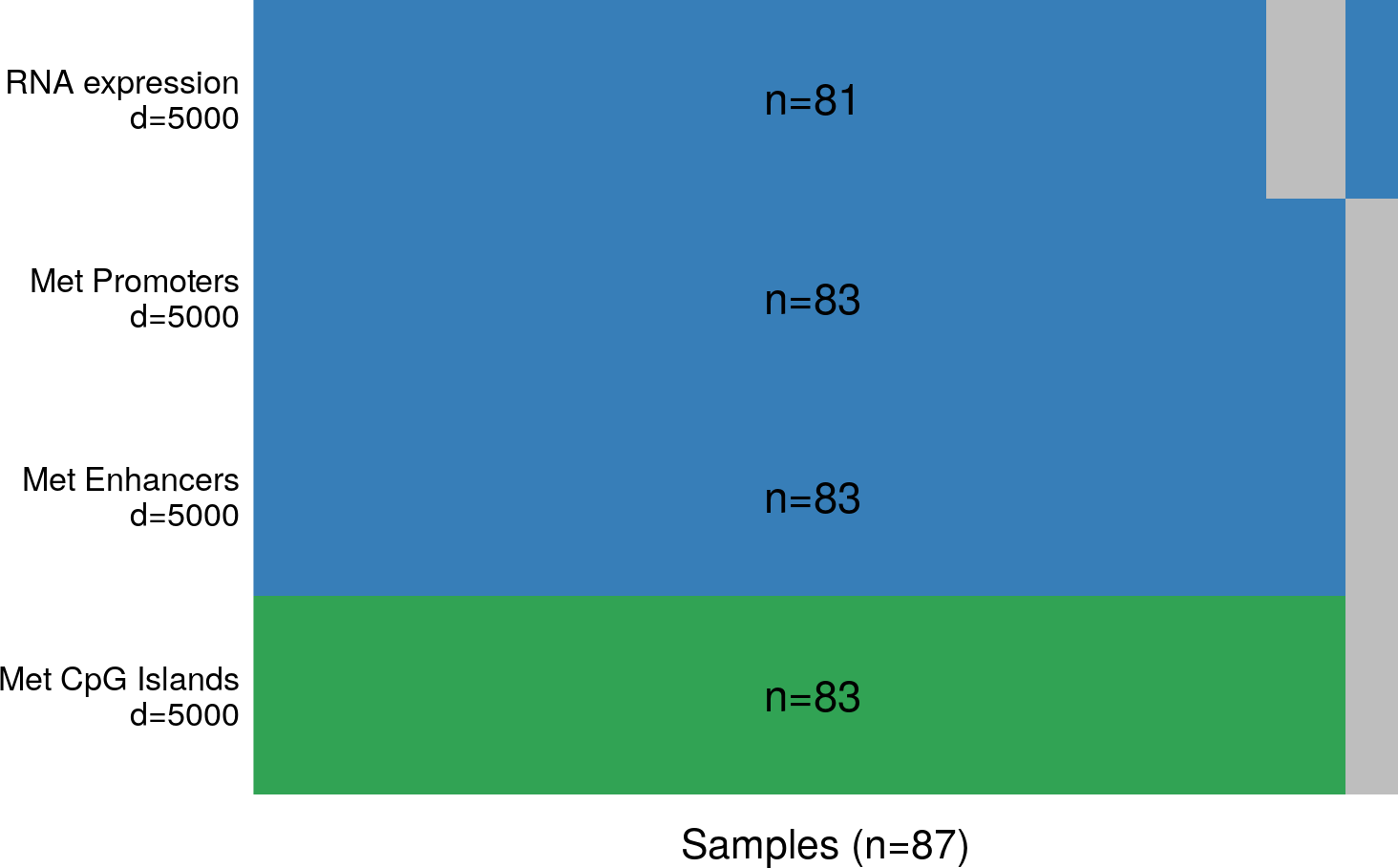# 2 Fit the MOFA model

The next step is to train the model. Internally, this is done via Python, so make sure you have the corresponding package installed (see installation instructions and read the FAQ if you have problems).

## 2.1 Define options

### 2.1.1 Define data options

Next, we define data options. The most important are:

• scaleViews: logical indicating whether to scale views to have unit variance. As long as the scale of the different data sets is not too high, this is not required. Default is `FALSE`.

• removeIncompleteSamples: logical indicating whether to remove samples that are not profiled in all omics. The model can cope with missing assays, so this option is not required. Default is `FALSE`.

``````DataOptions <- getDefaultDataOptions()
DataOptions``````
``````## \$scaleViews
##  FALSE
##
## \$removeIncompleteSamples
##  FALSE``````

### 2.1.2 Define model options

Next, we define model options. The most important are:

• numFactors: number of factors (default is 0.5 times the number of samples). By default, the model will only remove a factor if it explains exactly zero variance in the data. You can increase this threshold on minimum variance explained by setting `TrainOptions\$dropFactorThreshold` to a value higher than zero.

• likelihoods: likelihood for each view. Usually we recommend gaussian for continuous data, bernoulli for binary data and poisson for count data. By default, the model tries to guess it from the data.

• sparsity: do you want to use sparsity? This makes the interpretation easier so it is recommended (Default is `TRUE`).

``````ModelOptions <- getDefaultModelOptions(MOFAobject)
ModelOptions``````
``````## \$likelihood
##  RNA expression   Met Enhancers Met CpG Islands   Met Promoters
##      "gaussian"     "bernoulli"     "bernoulli"     "bernoulli"
##
## \$numFactors
##  44
##
## \$sparsity
##  TRUE``````

### 2.1.3 Define training options

Next, we define training options. The most important are:

• maxiter: maximum number of iterations. Ideally set it large enough and use the convergence criteria `TrainOptions\$tolerance`.

• tolerance: convergence threshold based on change in the evidence lower bound. For an exploratory run you can use a value between 1.0 and 0.1, but for a “final” model we recommend a value of 0.01.

• DropFactorThreshold: hyperparameter to automatically learn the number of factors based on a minimum variance explained criteria. Factors explaining less than ‘DropFactorThreshold’ fraction of variation in all views will be removed. For example, a value of 0.01 means that factors that explain less than 1% of variance in all views will be discarded. By default this it zero, meaning that all factors are kept unless they explain no variance at all. Here we use a threshold of 1%.

``````TrainOptions <- getDefaultTrainOptions()
TrainOptions\$seed <- 2018

# Automatically drop factors that explain less than 1% of variance in all omics
TrainOptions\$DropFactorThreshold <- 0.01

TrainOptions``````
``````## \$maxiter
##  5000
##
## \$tolerance
##  0.1
##
## \$DropFactorThreshold
##  0.01
##
## \$verbose
##  FALSE
##
## \$seed
##  2018``````

## 2.2 Prepare MOFA

`prepareMOFA` internally performs a set of sanity checks and fills the `DataOptions`, `TrainOptions` and `ModelOptions` slots of the `MOFAobject`

``````MOFAobject <- prepareMOFA(
MOFAobject,
DataOptions = DataOptions,
ModelOptions = ModelOptions,
TrainOptions = TrainOptions
)``````
``## Checking data options...``
``## Checking training options...``
``## Checking model options...``

## 2.3 Run MOFA

Now we are ready to train the `MOFAobject`, which is done with the function `runMOFA`. This step can take some time (around 5 min with default parameters for a single trial). For illustration we provide an existing trained `MOFAobject`

``MOFAobject <- runMOFA(MOFAobject)``
``````filepath <- system.file("extdata", "scMT_model.hdf5", package = "MOFAdata")
``##  "Could not load the feature intercepts. Your features will be centered to zero mean"``
``## Warning: Factor 3 is strongly correlated with the total expression for each sample in RNA expression``
``## Such (strong) factors usually appear when count-based assays are not properly normalised by library size.``
``MOFAobject``
``````## Trained MOFA model with the following characteristics:
##   Number of views: 4
##  View names: Met CpG Islands Met Enhancers Met Promoters RNA expression
##   Number of features per view: 5000 5000 5000 5000
##   Number of samples: 87
##   Number of factors: 3``````

# 3 Analyse a trained MOFA model

After training, we can explore the results from MOFA. Here we provide a semi-automated pipeline to disentangle and characterize all the identified sources of variation (the factors).

Part 1: Disentangling the heterogeneity

Calculation of variance explained by each factor in each view. This is probably the most important plot that MOFA generates, as it summarises the entire heterogeneity of the dataset in a single figure.

Part 2: Characterization of individual factors

• Feature set enrichment analysis (where set annotations are present, e.g. gene sets for mRNA views).
• Ordination of samples by factors to reveal clusters and/or gradients: this is similar to what is traditionally done with Principal Component Analysis or t-SNE.

Other analyses, including imputation of missing values and prediction of clinical data are also available. See below for a short guide on how to impute data. Detailed vignettes will follow soon.

## 3.1 Disentangling the heterogeneity, calculation of variance explained by each factor in each view

This is done by `calculateVarianceExplained` (to get the numerical values) and `plotVarianceExplained` (to get the plot).
The resulting figure gives an overview of which factors are active in which view(s). If a factor is active in more than one view, this means that is capturing shared signal (co-variation) between features of different data modalities.

In this data set MOFA identified 3 Factors with a minimum variance of 1%. While Factor 1 is shared across all data modalities (12% variance explained in the RNA data and between 53% and 72% in the methylation data sets), Factors 2 and 3 are active primarily in the RNA data

``````# Calculate the variance explained (R2) per factor in each view
r2 <- calculateVarianceExplained(MOFAobject)
r2\$R2Total``````
``````## Met CpG Islands   Met Enhancers   Met Promoters  RNA expression
##       0.5685838       0.7284391       0.5277814       0.1231761``````
``````# Variance explained by each factor in each view
``````##     Met CpG Islands Met Enhancers Met Promoters RNA expression
## LF1    5.683954e-01  7.282991e-01  0.5272673784     0.06255281
## LF2    1.178354e-04  8.761564e-05  0.0003002201     0.03548858
## LF3    9.120354e-05  9.245025e-05  0.0002861076     0.03239565``````
``````# Plot it
plotVarianceExplained(MOFAobject)``````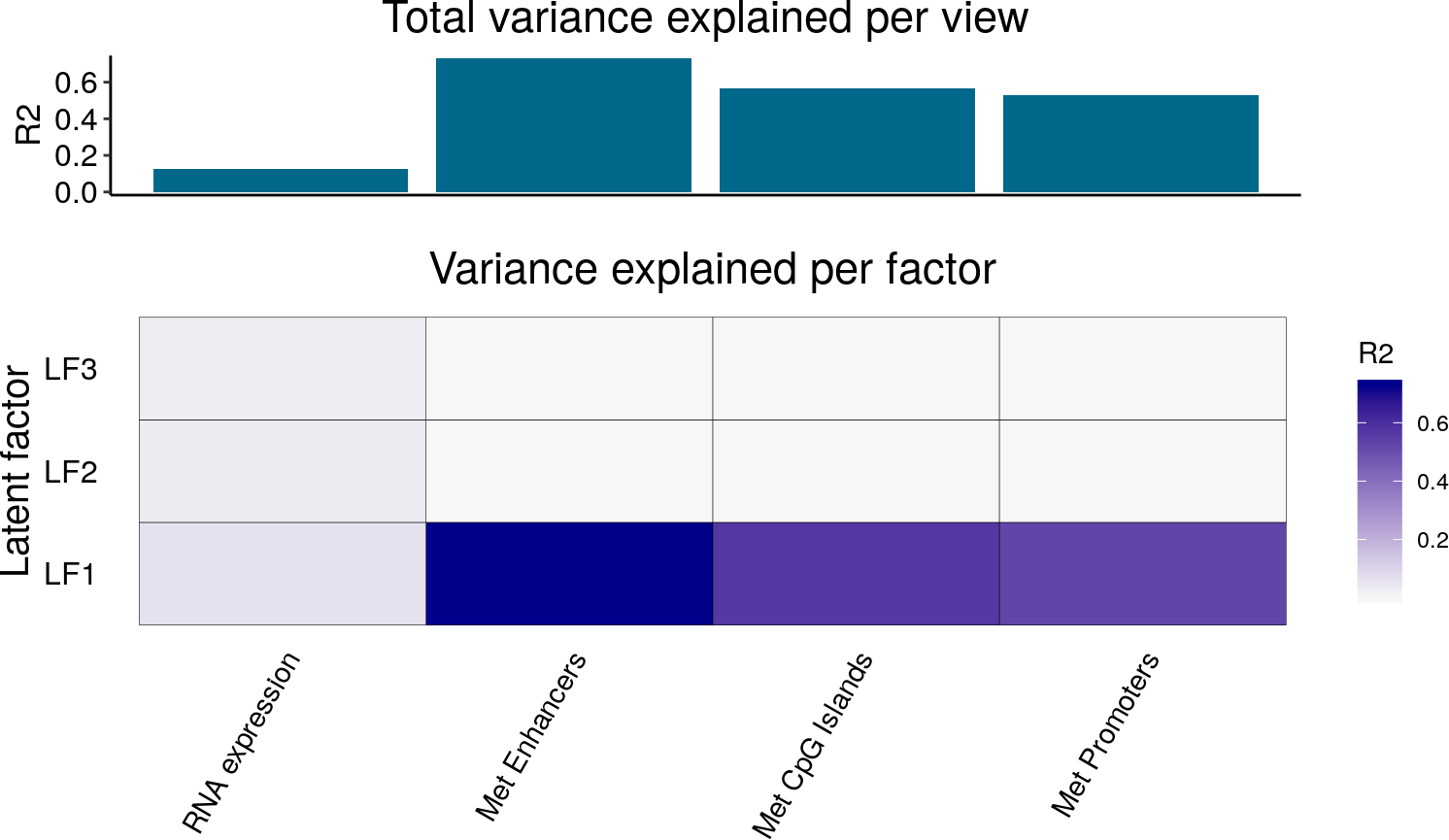## 3.2 Characterisation of individual factors

Plotting the RNA expression gene loadings for Factor 1, we can see that it is enriched for naive pluripotency marker genes such as Rex1/Zpf42, Tbx3, Fbxo15 and Essrb. Hence, based on previous studies (Mohammed et al, 2016) we can hypothesise that Factor 1 captures a transition from a naive pluripotent state to a primed pluripotent states.

``````# Plot all weights and highlight specific gene markers
plotWeights(
object = MOFAobject,
view = "RNA expression",
factor = 1,
nfeatures = 0,
abs = FALSE,
manual = list(c("Zfp42","Esrrb","Morc1","Fbxo15",
"Jam2","Klf4","Tcl1","Tbx3","Tex19.1"))
)``````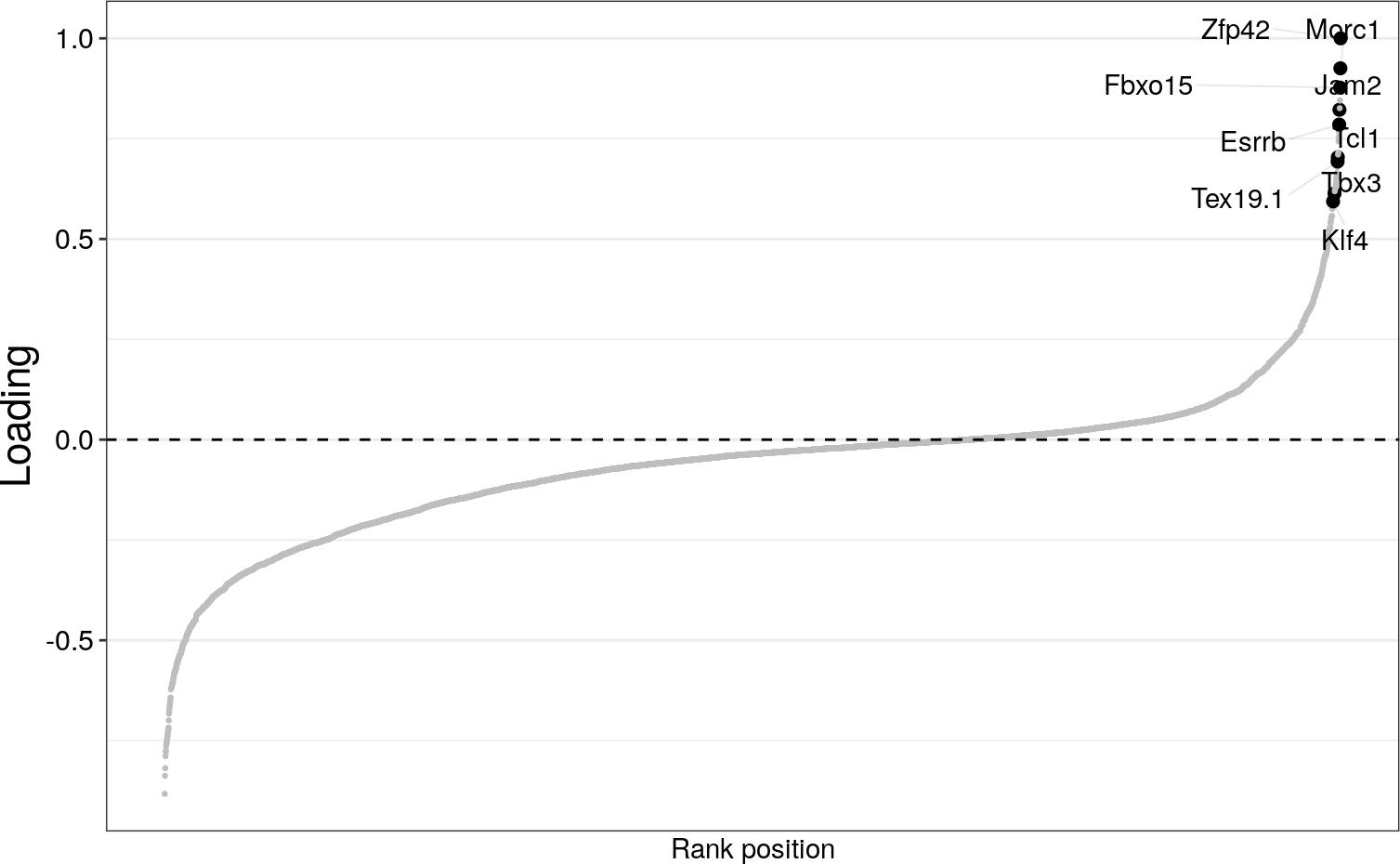``````# Plot top 10 genes
plotTopWeights(
object = MOFAobject,
view = "RNA expression",
factor = 1,
nfeatures = 10
)``````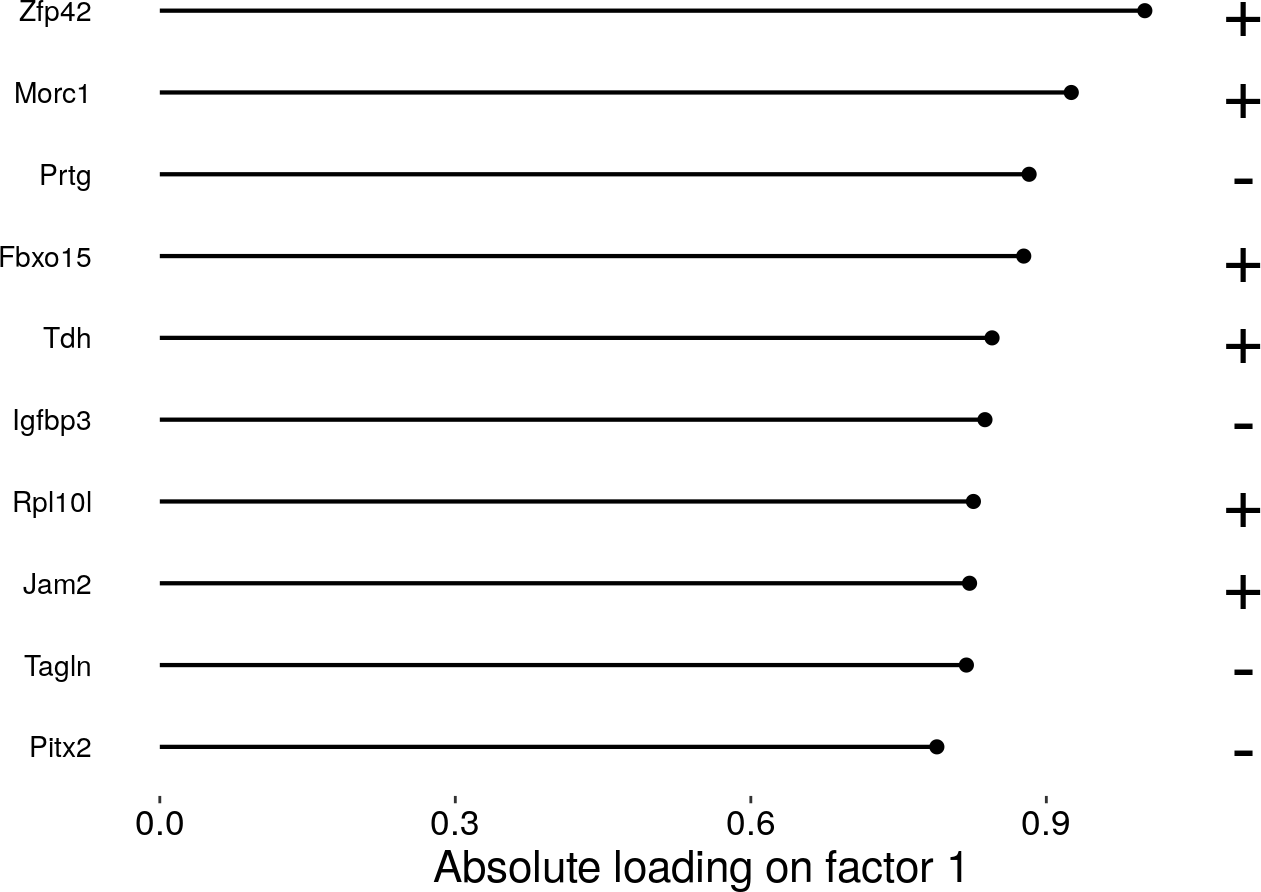Also, instead of looking at the “abstract” weights, it is useful to observe, in the original data, the heterogeneity captured by a Factor. This can be done using the `plotDataHeatmap` function.

``````# Add metadata to the plot
factor1 <- sort(getFactors(MOFAobject,"LF1")[,1])
order_samples <- names(factor1)
df <- data.frame(
row.names = order_samples,
culture = getCovariates(MOFAobject, "culture")[order_samples],
factor = factor1
)

plotDataHeatmap(
object = MOFAobject,
view = "RNA expression",
factor = "LF1",
features = 20,
transpose = TRUE,
show_colnames=FALSE, show_rownames=TRUE, # pheatmap options
cluster_cols = FALSE, annotation_col=df # pheatmap options
)``````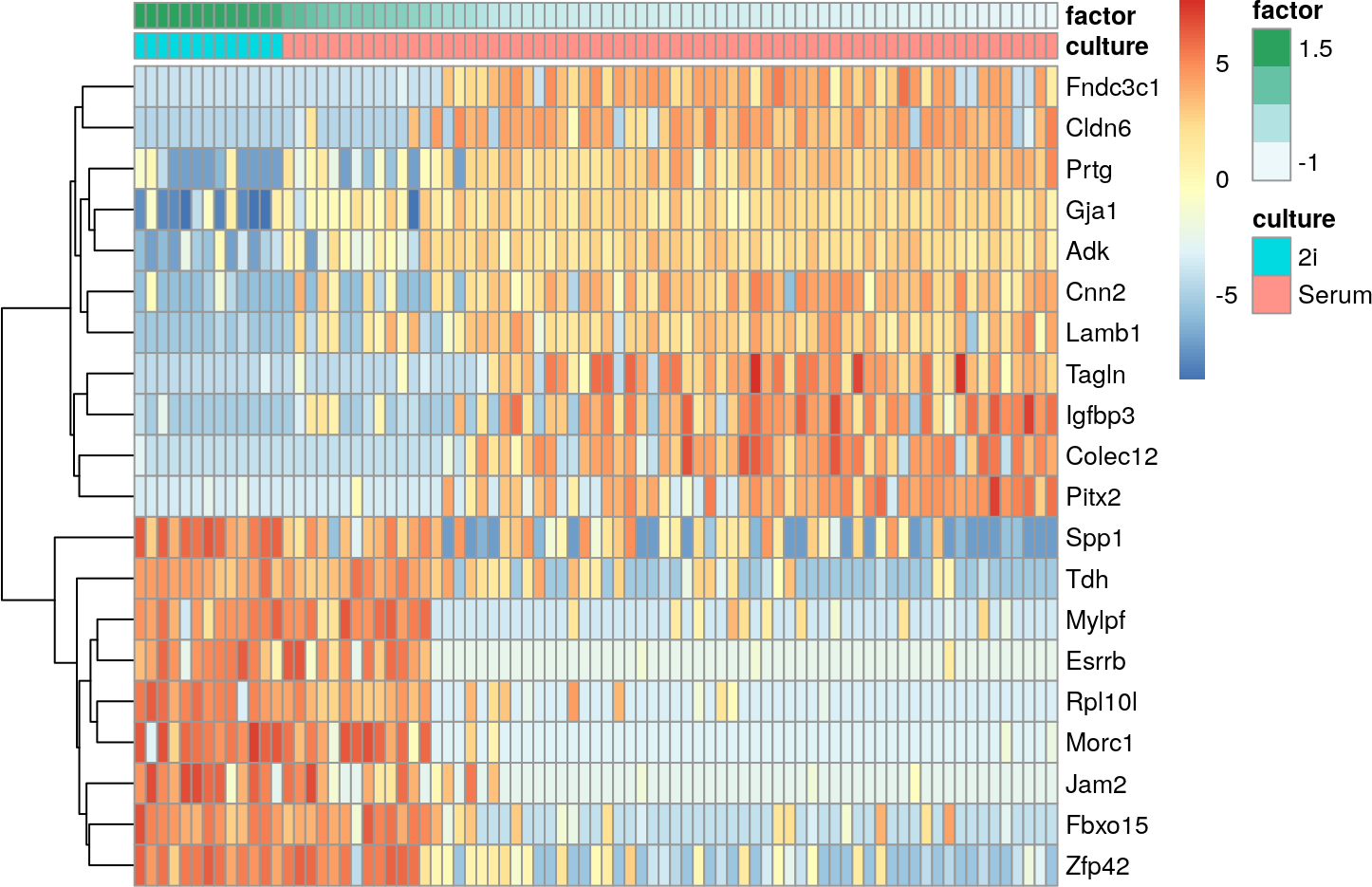We can now connect these transcriptomic changes to coordinated changes on the DNA methylation. As Factor 1 is active in all genomic contexts, it suggests that there is a massive genome-wide DNA methylation remodelling. This can confirmed by inspecting the weights in the DNA methylation views, as we do here for the CpG sites in enhancers: Notice that most features (CpG sites) have a negative weight, which suggests that their DNA methylation decrease in a manner that is inversely proportional to the direction of Factor 1.

``````plotWeights(
object = MOFAobject,
view = "Met Enhancers",
factor = 1,
nfeatures = 0,
abs = FALSE,
scale = FALSE
)``````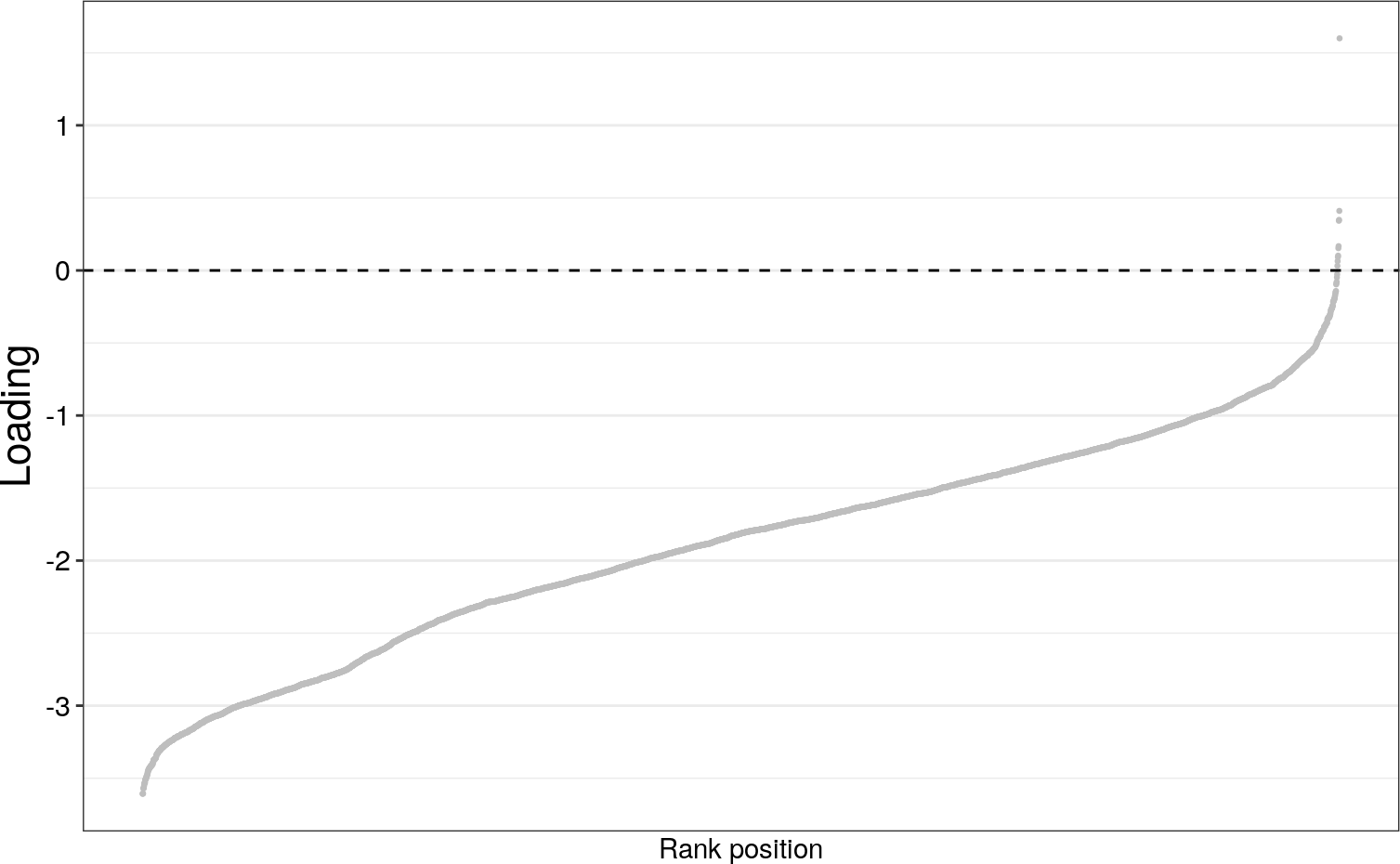Similar observations can be made for the CpG sites in other genomic contexts, here CpG Islands or Promotors, by plotting the weights of the respective views (i.e. “Met CpG Islands” or “Met Promoters”).

As done before, let’s observe the heterogeneity captured by Factor 1 in the original data space. This clearly confirms that most of the CpG sites are getting methylated as cells progress in Factor 1 from naive to primed pluripotent stem cells

``````plotDataHeatmap(
object = MOFAobject,
view = "Met Enhancers",
factor = 1,
features = 500,
transpose = TRUE,
cluster_rows=FALSE, cluster_cols=FALSE, # pheatmap options
show_rownames=FALSE, show_colnames=FALSE, # pheatmap options
annotation_col=df  # pheatmap options
)``````